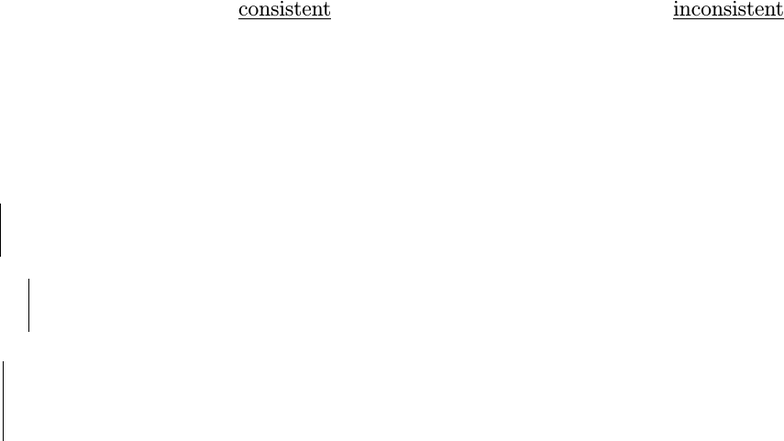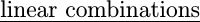Study Guides (380,000)
US (220,000)
UCLA (3,000)
MATH (300)
All (20)
Final

MATH 33AH Lecture Notes - Lecture 9: Free Variables And Bound Variables, Linear Combination, Elementary MatrixExam

Department
Mathematics
Course Code
MATH 33AH
Professor
All
Study Guide
Final

This preview shows pages 1-3. to view the full 10 pages of the document.Math 33A Discussion Notes
Jean-Michel Maldague
October 20, 2017
Week 2
- We want to do more with linear systems, so to this end we discuss matrices.
- In the back of our minds, we still often think of matrices as coeﬃcient matrices of an
associated linear system.
- Today: properties of and operations on matrices.
rank(A) = # of pivots in rref(A)
- If A is a coeﬃcient matrix in some linear system, this means:
rank(A) = # of leading vars = # vars # free vars,
since each column is either a pivot column or a free variable column.
- Each row and column can house at most one pivot, by the rref requirements. So if A is
of size n×k, then rank(A)min(n,k).
- Terminology: a linear system is consistent if there is at least one solution; it is inconsistent
if there are no solutions.
- Given a system that has been put into rref, we may quickly determine the state of aﬀairs...
a) The following systems are in the form rref([ A|~
b]), where A is the coeﬃcient matrix
and ~
bis the column of constants. Which are consistent and which are inconsistent? For
those that are consistent, are there inﬁnitely many solutions, or exactly one solution?
1 0 0
0 1 7(consistent, since we don’t get 0 = 1. 1 soln, since there are no free variables)
1 0 0 0
0 1 2 4(consistent, since we don’t get 0 = 1. solns, since there is a free variable)
1 0 0
0 1 0
0 0 1
(inconsistent, since the last line reads 0 = 1)
1

Only pages 1-3 are available for preview. Some parts have been intentionally blurred.1 0 0
0 1 3
0 0 0
(consistent, since we don’t get 0 = 1. 1 soln, since there are no free variables).
////
- Note that the system is inconsistent iﬀ (“if and only if”) the rank of the augmented ma-
trix is 1 more than the rank of the coeﬃcient matrix. This is clearly the case in the above
examples. The reason for this is the last column can house at most one pivot, and if there
is a pivot, then there are only zeros to the left (in the rref of the coeﬃcient matrix), so
we get 0 = 1. If there are no pivots in the last column, then every nonzero element in it
has a pivot to its left (meaning, a pivot in the same row, in the rref of the coeﬃcient matrix).
- We now discuss several useful operations on matrices. For this section, we’ll use the
following notation: if C is a matrix of size nxk and 1 in, 1jk, then Ci,j is the
(i,j)th entry of C (so, the entry in the ith row and jth column of C, counting from the top left).
- Matrix addition is componentwise, and requires matrices to have the same size. The
formula is: (A+B)i,j =Ai,j +Bi,j . i.e.:
1 2 3
4 5 6 +789
10 11 12 =8 10 12
14 16 18 .
- Scalar multiplication is just as easy: everything in the matrix gets multiplied by the same
constant. (kC)i,j =k(Ci,j). i.e.
k1 2
3 4 =k2k
3k4k.
- Matrix multiplication is weird (for now; it makes sense later). The inner dimensions must
agree for the matrix product to be deﬁned. If A is size n×k and B is size k×p (so A has the
same number of columns as B has rows), then the matrix product is given by:
(AB)i,j =
k
X
l=1
Ai,lBl,j.
The matrix product will be of size n×p (imagine the inner dimensions collapsing). e.g.
1 2 3
4 5 6
7 10 13 16
8 11 14 17
9 12 15 18
=50 68 86 104
122 167 212 257
- The transpose of a matrix A is just the matrix with all of its rows and columns ﬂipped:
(AT)i,j =Aj,i ,
2

Only pages 1-3 are available for preview. Some parts have been intentionally blurred.i.e.
123
456
789
10 11 12
T
=
1 4 7 10
2 5 8 11
3 6 9 12
.
- The dot product of two column vectors ~v, ~w Rnis:
~v ·~w =~v T~w =
n
X
i=1
viwi.
- There are three important interpretations of matrix multiplication, but to get to them we
will ﬁrst need another deﬁnition.
If ~v1, . . . , ~vkare vectors, then expressions of the form c1~v1+· · · ck~vkare called linear combinations
of the ~vi’s, using the ci’s as weights.
˜˜˜ Three Interpretations of Matrix Multiplication ˜˜˜
matrix * vector is a linear combination of the matrix’s columns, using the vector’s
entries as weights: if ~v1, . . . ~vkRnare column vectors and x1, . . . , xkR, then
[~v1. . . ~vk]
x1
.
.
.
xk
=x1~v1+· · · +xk~vk
(i,j)th entry of AB is ith row of A dotted with jth column of B: if ~v1, . . . , ~vnRkand
~w1, . . . , ~wpRk, then:
~v T
1
.
.
.
~v T
n
~w1. . . ~wp=
~v1·~w1· · · ~v1·~wp
.
.
.....
.
.
~vn·~w1· · · ~vn·~wp
row vec * matrix is a linear combination of the matrix’s rows, using the vector’s entries
as weights: if x1, . . . , xkRand ~v1, . . . , ~vkRp, then
[x1, . . . , xk]
~v T
1
.
.
.
~v T
k
=x1~v T
1+· · · +xk~v T
k.
3